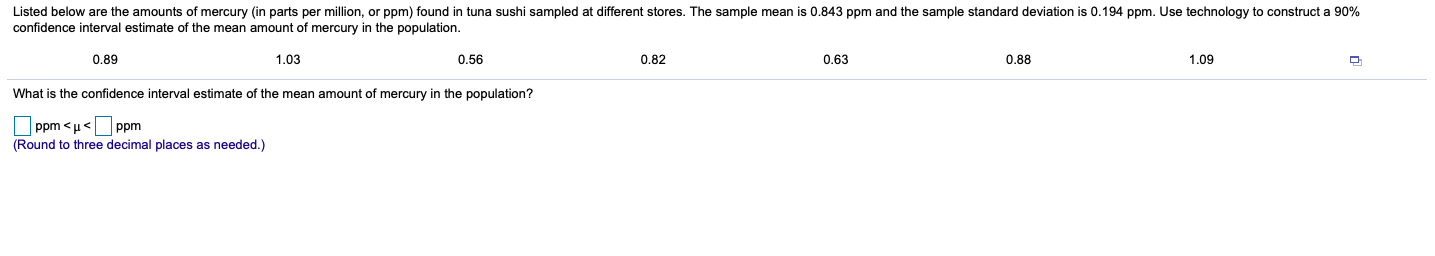# Listed below are the amounts of mercury (in parts per million, or ppm) found in tuna sushi sampled at different stores. The sample mean is 0.843 ppm and the sample standard deviation is 0.194 ppm. Use technology to construct a 90% confidence interval estimate of the mean amount of mercury in the population. 0.89 1,03 0.56 0.82 0.63 0.88 1,09 What is the confidence interval estimate f the mean amount of mercury in the population? Ppm < u

Question
Listed below are the amounts of mercury​ (in parts per​ million, or​ ppm) found in tuna sushi sampled at different stores. The sample mean is
0.843ppm and the sample standard deviation is 0.194ppm. Use technology to construct a 90​% confidence interval estimate of the mean amount of mercury in the population.help_outlineImage TranscriptioncloseListed below are the amounts of mercury (in parts per million, or ppm) found in tuna sushi sampled at different stores. The sample mean is 0.843 ppm and the sample standard deviation is 0.194 ppm. Use technology to construct a 90% confidence interval estimate of the mean amount of mercury in the population. 0.89 1,03 0.56 0.82 0.63 0.88 1,09 What is the confidence interval estimate f the mean amount of mercury in the population? Ppm < u

1 Rating

### Want to see this answer and more?

Experts are waiting 24/7 to provide step-by-step solutions in as fast as 30 minutes!*